# imflatfield

2-D image flat-field correction

## Syntax

J = imflatfield(I,sigma)
J = imflatfield(I,sigma,mask)
J = imflatfield(___,'FilterSize',filterSize)

## Description

example

J = imflatfield(I,sigma) applies flat-field correction to the grayscale or RGB image I. The correction uses Gaussian smoothing with a standard deviation of sigma to approximate the shading component of I. The corrected image is returned in J.

example

J = imflatfield(I,sigma,mask) applies flat-field correction to image I only where the binary mask is true. Where the mask is false, the output image J contains the unmodified values of image I.
J = imflatfield(___,'FilterSize',filterSize) specifies the size of the Gaussian smoothing filter.

## Examples

collapse all

Load a grayscale image. This image has severe shading distortion on the left side and in the upper-right corner.

I = imread('printedtext.png'); imshow(I) title('Distorted Image')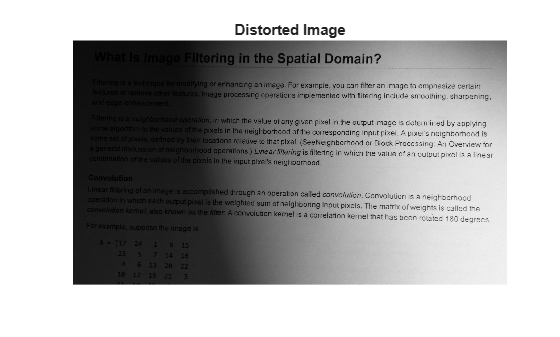Perform the flat-field correction.

sigma = 30; Iflatfield = imflatfield(I,sigma);

Display the result. The corrected image has more uniform brightness.

imshow(Iflatfield) title(['Flat-Field Corrected Image, \sigma = ',num2str(sigma)])Load a color image that has vignetting, or darkening of the corners.

I = imread('fabric.png'); imshow(I) title('Image with Vignetting')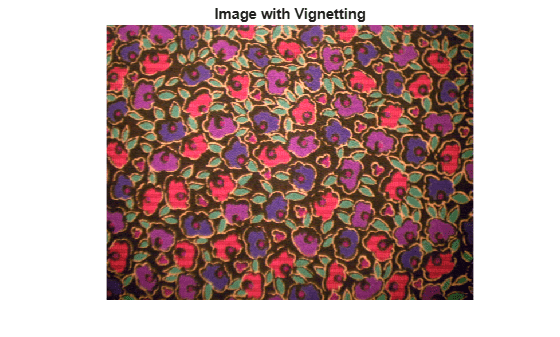Perform the flat-field correction.

sigma = 20; Iflatfield = imflatfield(I,sigma);

Display the result. The corrected image has more uniform brightness.

imshow(Iflatfield) title(['Flat-Field Corrected Image, \sigma = ',num2str(sigma)])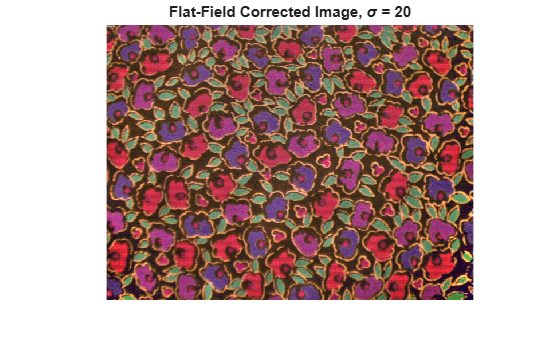Load a color image. This image has a shading defect in the lower right corner.

I = imread('hands1.jpg'); imshow(I) title('Image with Dark Corner')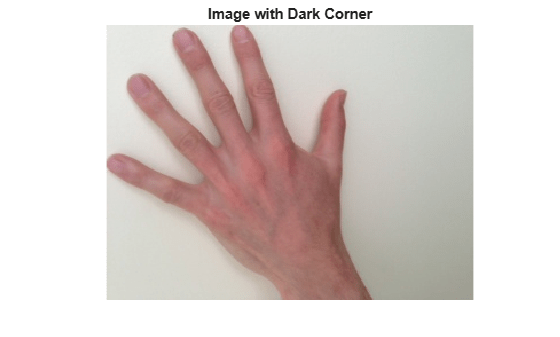Try applying flat-field correction to the entire image.

sigma = 25; Iflatfield = imflatfield(I,25); imshow(Iflatfield) title(['Flat-Field Corrected Image, \sigma = ',num2str(sigma)])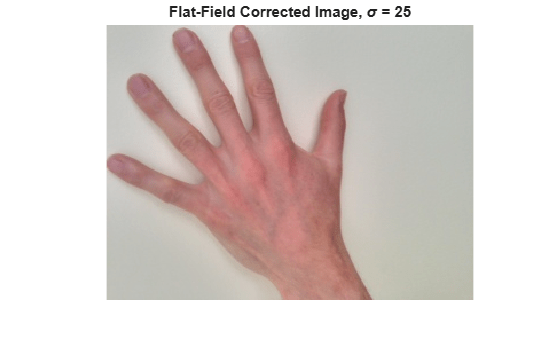The shading defect in the corner is corrected, but the center of the image is too bright and the hand has changed color. To avoid this brightening artifact, apply flat-field correction just to the background of the image.

Load the mask of this image. In the original mask, maskHand, the segmented hand is the region of interest (ROI). Invert the mask so that the background is the ROI. Display the mask, which shows the ROI in white.

maskHand = imread('hands1-mask.png'); maskBackground = ~maskHand; imshow(maskBackground) title('Background Mask')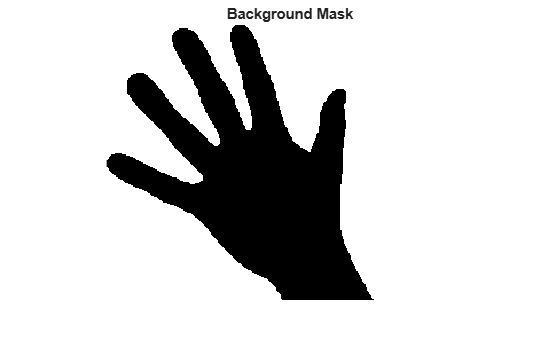Perform the flat-field correction on the background of the image using the mask maskBackground. The hand is not a region of interest in the mask, therefore flat-field correction is not applied to pixels in the hand.

Iflatfield2 = imflatfield(I,sigma,maskBackground);

Display the corrected image. The shading defect in the corner is corrected, and the hand retains its original color.

imshow(Iflatfield2) title(['Flat-Field Corrected Background, \sigma = ',num2str(sigma)])## Input Arguments

collapse all

Distorted image, specified as a 2-D grayscale image of size m-by-n or a 2-D RGB image of size m-by-n-by-3.

Data Types: single | double | int16 | uint8 | uint16

Standard deviation of the Gaussian smoothing filter, specified as a positive number or a 2-element vector of positive numbers. If you specify a scalar, then imflatfield uses a square Gaussian kernel.

Data Types: single | double | int8 | int16 | int32 | int64 | uint8 | uint16 | uint32 | uint64

Binary mask, specified as a 2-D numeric or logical matrix of size m-by-n. For numeric input, any nonzero pixels are considered to be 1 (true).

Data Types: single | double | int8 | int16 | int32 | int64 | uint8 | uint16 | uint32 | uint64

Size of the Gaussian filter, specified as a scalar or 2-element vector of positive, odd integers. If you specify a scalar, then imflatfield uses a square filter. The default filter size is 2*ceil(2*sigma)+1.

Data Types: single | double | int8 | int16 | int32 | int64 | uint8 | uint16 | uint32 | uint64

## Output Arguments

collapse all

Corrected image, returned as a 2-D grayscale or RGB image of the same size and data type as the input image, I.

## Tips

• When I is an RGB image, then imflatfield converts the image to the HSV color space using rgb2hsv and applies the flat-field correction to the HSV Value channel. The image is converted back to RGB color space by using hsv2rgb.

• If you specify a mask, then imflatfield dilates the mask and pads the image boundaries to reduce edge artifacts during the flat-field estimation.